# 基于Spark的并行化出租车轨迹热点区域提取与分析Extraction and Analysis of Hotspot Region of Parallel Taxi Trajectory Based on Spark

DOI: 10.12677/CSA.2018.89161, PDF, HTML, XML, 下载: 679  浏览: 4,029  国家自然科学基金支持

Abstract: The taxi GPS trajectory data can mine wealthy residents travel law information, but for the increasing number of data, there are new requirements have been put forward about the accuracy and efficiency of data mining. This paper takes Chengdu taxi GPS trajectory data as the research object. First, the distortion of the original data and the redundant field should be deleted, and partial time data should be filtered, then the map should be matched; finally using the spark Big Data processing platform, it realized K-means| |, divided into working days and rest days to analyze and get the hot spot area of Chengdu residents and its space-time distribution characteristics. Finally, com-pared the performance of the K-means and K-means| |, the result showed that K-means| | had superiority in accuracy and time efficiency compared with the single machine.

1. 引言

2. 数据预处理

2.1. 失真数据剔除

2.2. 多余字段删除

2.3. 部分时段数据过滤

00:00:00~05:59:59时间段出租车基本处于停运状态，该时间段的轨迹数据对于提取居民出行高峰时段和挖掘分析城市热点区域没有参考价值，因此删除这段时间的轨迹数据。

3. 地图匹配

$S=q{\sum }_{i=0}^{n}{D}_{i}$ (1)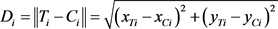(2)

4. 基本原理与方法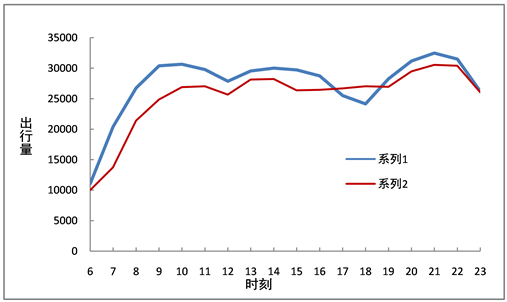Figure 1. Comparison of travel volume of residents in each period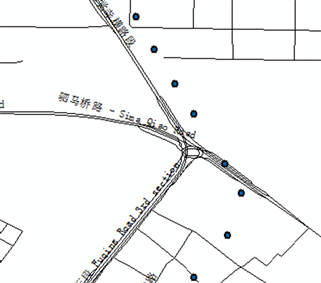(a)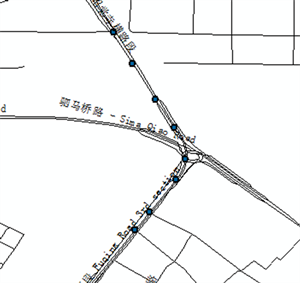(b)

Figure 2. The comparison of before and after map matching. (a) Before the matched map; (b) After the matched map

$\begin{array}{l}\text{SqDist}={\left(\sqrt{{a}_{1}^{2}+{b}_{1}^{2}}-\sqrt{{a}_{2}^{2}+{b}_{2}^{2}}\right)}^{2}\\ \text{\hspace{0.17em}}\text{\hspace{0.17em}}\text{\hspace{0.17em}}\text{\hspace{0.17em}}\text{\hspace{0.17em}}\text{\hspace{0.17em}}\text{\hspace{0.17em}}\text{\hspace{0.17em}}\text{\hspace{0.17em}}={a}_{1}^{2}+{b}_{1}^{2}+{a}_{2}^{2}+{b}_{2}^{2}-2\sqrt{\left({a}_{1}^{2}+{b}_{1}^{2}\right)\left({a}_{2}^{2}+{b}_{2}^{2}\right)}\end{array}$ (3)

5. 实验与分析Table 1. K-Means|| detailed parameters

5.1. 城市热点提取

$d{h}_{i}=\frac{{n}_{i}}{m}$ , (4)

5.2. K-Means||算法性能分析Table 2. Distribution of early peak hotspots on August 4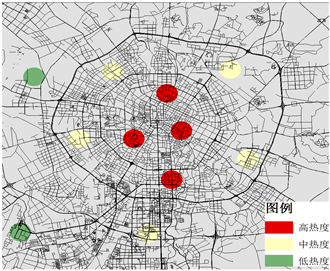(a)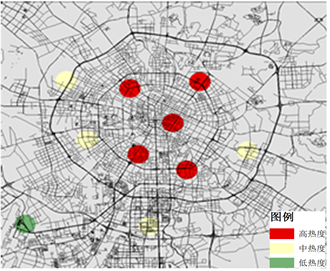(b)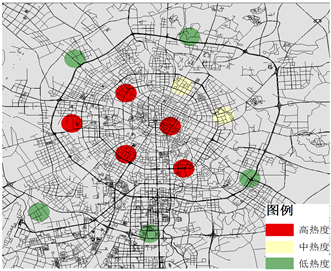(c)

Figure 3. Distribution of hotspots during peak hours on workday. (a) Early peak distribution; (b) Midday peak distribution; (c) Late peak distribution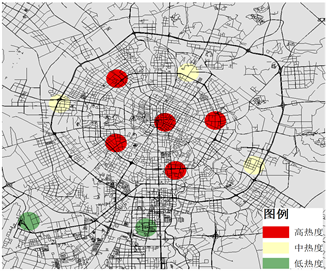(a)(b)

Figure 4. Distribution of hotspots during peak hours on weekend. (a) Midday peak distribution; (b) Late peak distribution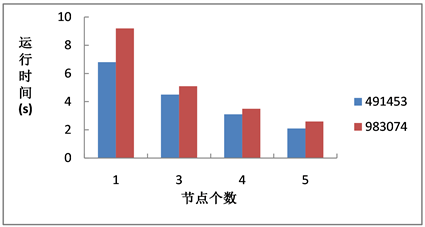Figure 5. Comparison of running time of different nodes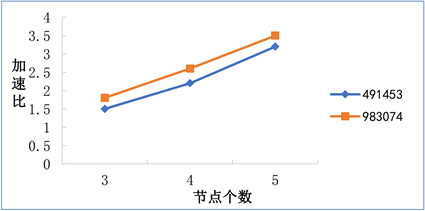Figure 6. Acceleration ratio of different nodes

$sq={t}_{1}/{t}_{n}$ (5)

6. 结束语

  Yue, Y., Wang, H.D., Hu, B., et al. (2012) Exploratory Calibration of a Spatial Interaction Model Using Taxi GPS Trajectories. Com-puters, Environment and Urban Systems, 36, 140-153. https://doi.org/10.1016/j.compenvurbsys.2011.09.002  Peng, C.B., Jin, X.G., Wong, K.C., Shi, M.X. and Pietro, L. (2012) Collective Human Mobility Pattern from Taxi Trips in Urban Area. PLoS One, 7. https://doi.org/10.1371/journal.pone.0034487  Veloso, M., Phithakkitnukoon, S. and Bento, C. (2011) Urban Mobility Study Using Taxi Traces. International Workshop on Trajectory Data Mining and Analysis, 23-30.  周勍, 秦昆, 陈一祥, 李志鑫. 基于数据场的出租车轨迹热点区域探测方法[J]. 地理与地理信息科学, 2016, 32(6): 51-56, 127.  张俊涛, 武芳, 张浩. 利用出租车轨迹数据挖掘城市居民出行特征[J]. 地理与地理信息科学, 2015, 31(6): 104-108.  Savage, N.S., Nishimura, S., Chavez, N.E., et al. (2010) Frequent Trajectory Mining on GPS Data. Proceedings of LocWeb, ACM Press, New York, 3-7.  付鑫, 孙茂棚, 孙皓. 基于GPS数据的出租车通勤识别及时空特征分析[J]. 中国公路学报, 2017, 30(7): 134-143.  程静, 刘家骏, 高勇. 基于时间序列聚类方法分析北京出租车出行量的时空特征[J]. 地球信息科学学报, 2016, 18(9): 1227-1239.  牟乃夏, 张恒才, 陈洁, 张灵先, 戴洪磊. 轨迹数据挖掘城市应用研究综述[J]. 地球信息科学学报, 2015, 17(10): 1136-1142.  桂智明, 向宇, 李玉鉴. 基于出租车轨迹的并行城市热点区域发现[J]. 华中科技大学学报(自然科学版), 2012, 40(S1): 187-190.  王丽鲲. 基于社交媒体地理数据挖掘的游客时空行为分析[D]: [硕士学位论文]. 上海: 上海师范大学, 2017.  葛小三, 付魁, 程钢, 马勇, 孙玉祥. 数据挖掘支持下的网络热点事件地理可视化研究[J]. 河南理工大学学报(自然科学版), 2016, 35(5): 655-659.  张玉峰, 曾奕棠. 基于动态数据挖掘的物流信息分析模型研究[J]. 情报科学, 2016, 34(1): 15-19, 33.  胡继华, 邓俊, 黄泽. 一种基于乘客出行轨迹的公交断面客流估算方法[J]. 计算机应用研究, 2014, 31(5): 1399-140.  毛峰. 基于多源轨迹数据挖掘的居民通勤行为与城市职住空间特征研究[D]: [博士学位论文]. 上海: 华东师范大学, 2015.